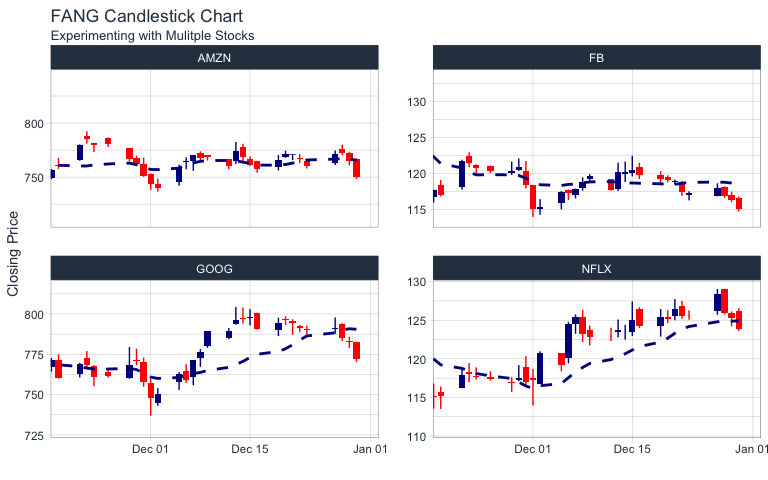# Charting with tidyquant

#### 2022-09-08

Charting financial data using `ggplot2`

# Overview

The `tidyquant` package includes charting tools to assist users in developing quick visualizations in `ggplot2` using the grammar of graphics format and workflow. There are currently three primary geometry (geom) categories and one coordinate manipulation (coord) category within `tidyquant`:

• Chart Types: Two chart type visualizations are available using `geom_barchart` and `geom_candlestick`.
• Moving Averages: Seven moving average visualizations are available using `geom_ma`.
• Bollinger Bands: Bollinger bands can be visualized using `geom_bbands`. The BBand moving average can be one of the seven available in Moving Averages.
• Zooming in on Date Ranges: Two `coord` functions are available (`coord_x_date` and `coord_x_datetime`), which prevent data loss when zooming in on specific regions of a chart. This is important when using the moving average and Bollinger band geoms.

# Prerequisites

Load the `tidyquant` package to get started.

``````# Loads tidyquant, lubridate, xts, quantmod, TTR, and PerformanceAnalytics
library(tidyverse)
library(tidyquant)  ``````

The following stock data will be used for the examples. Use `tq_get` to get the stock prices.

``````# Use FANG data set
data("FANG")

# Get AAPL and AMZN Stock Prices
AAPL <- tq_get("AAPL", get = "stock.prices", from = "2015-09-01", to = "2016-12-31")
AMZN <- tq_get("AMZN", get = "stock.prices", from = "2000-01-01", to = "2016-12-31")``````

The `end` date parameter will be used when setting date limits throughout the examples.

``````end <- as_date("2016-12-31")

end``````
``##  "2016-12-31"``

The `AAPL_range_60` will be used to adjust the zoom on the plots.

``````aapl_range_60_tbl <- AAPL %>%
tail(60) %>%
summarise(
max_high = max(high),
min_low  = min(low)
)

aapl_range_60_tbl``````
``````## # A tibble: 1 × 2
##   max_high min_low
##      <dbl>   <dbl>
## 1     29.7    26.0``````

# Chart Types

Financial charts provide visual cues to open, high, low, and close prices. The following chart geoms are available:

## Line Chart

Before we visualize bar charts and candlestick charts using the `tidyquant` geoms, let’s visualize stock prices with a simple line chart to get a sense of the “grammar of graphics” workflow. This is done using the `geom_line` from the `ggplot2` package. The workflow begins with the stock data, and uses the pipe operator (`%>%`) to send to the `ggplot()` function.

The primary features controlling the chart are the aesthetic arguments: these are used to add data to the chart by way of the `aes()` function. When added inside the `ggplot()` function, the aesthetic arguments are available to all underlying layers. Alternatively, the aesthetic arguments can be applied to each geom individually, but typically this is minimized in practice because it duplicates code. We set aesthetic arguments, `x = date` and `y = close`, to chart the closing price versus date. The `geom_line()` function inherits the aesthetic arguments from the `ggplot()` function and produces a line on the chart. Labels are added separately using the `labs()` function. Thus, the chart is built from the ground up by starting with data and progressively adding geoms, labels, coordinates / scales and other attributes to create a the final chart. This is enables maximum flexibility wherein the analyst can create very complex charts using the “grammar of graphics”.

``````AAPL %>%
ggplot(aes(x = date, y = close)) +
geom_line() +
labs(title = "AAPL Line Chart", y = "Closing Price", x = "") +
theme_tq()``````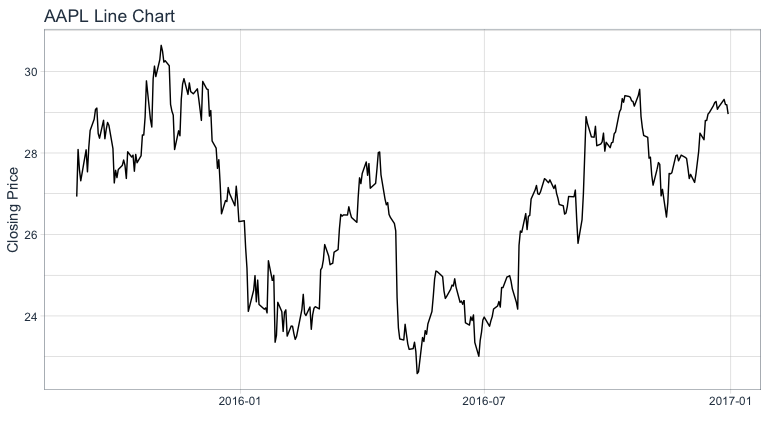## Bar Chart

Visualizing the bar chart is as simple as replacing `geom_line` with `geom_barchart` in the ggplot workflow. Because the bar chart uses open, high, low, and close prices in the visualization, we need to specify these as part of the aesthetic arguments, `aes()`. We can do so internal to the geom or in the `ggplot()` function.

``````AAPL %>%
ggplot(aes(x = date, y = close)) +
geom_barchart(aes(open = open, high = high, low = low, close = close)) +
labs(title = "AAPL Bar Chart", y = "Closing Price", x = "") +
theme_tq()``````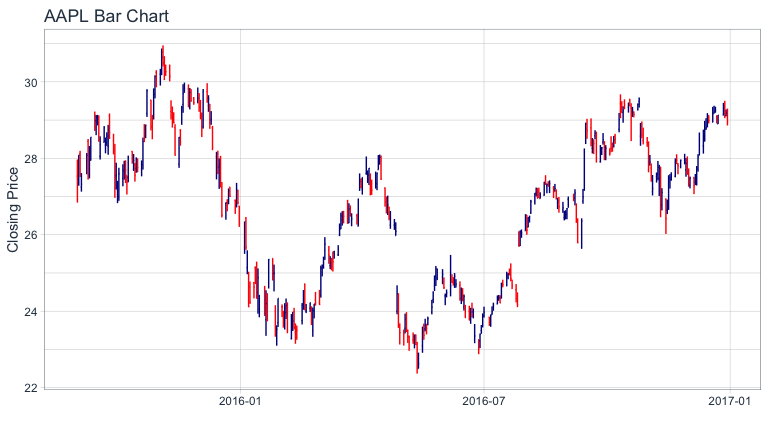We zoom into specific sections using `coord_x_date`, which has `xlim` and `ylim` arguments specified as `c(start, end)` to focus on a specific region of the chart. For `xlim`, we’ll use `lubridate` to convert a character date to date class, and then subtract six weeks using the `weeks()` function. For `ylim` we zoom in on prices in the range from 100 to 120.

``````AAPL %>%
ggplot(aes(x = date, y = close)) +
geom_barchart(aes(open = open, high = high, low = low, close = close)) +
labs(title = "AAPL Bar Chart",
subtitle = "Zoomed in using coord_x_date",
y = "Closing Price", x = "") +
coord_x_date(xlim = c(end - weeks(6), end),
ylim = c(aapl_range_60_tbl\$min_low, aapl_range_60_tbl\$max_high)) +
theme_tq()``````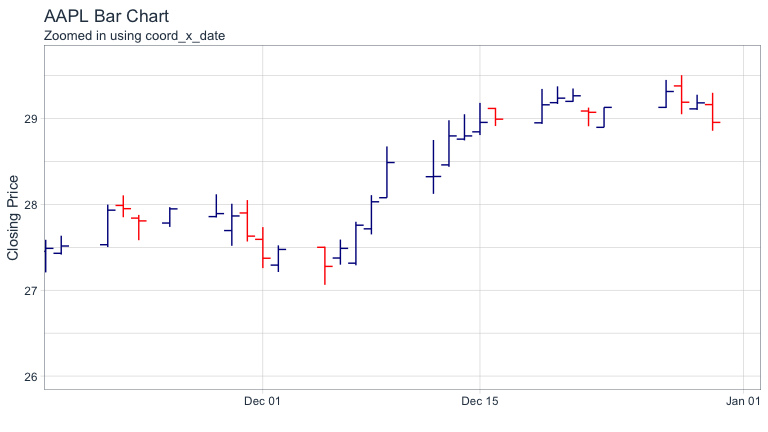The colors can be modified using `colour_up` and `colour_down` arguments, and parameters such as `size` can be used to control the appearance.

``````AAPL %>%
ggplot(aes(x = date, y = close)) +
geom_barchart(aes(open = open, high = high, low = low, close = close),
colour_up = "darkgreen", colour_down = "darkred", size = 1) +
labs(title = "AAPL Bar Chart",
subtitle = "Zoomed in, Experimenting with Formatting",
y = "Closing Price", x = "") +
coord_x_date(xlim = c(end - weeks(6), end),
c(aapl_range_60_tbl\$min_low, aapl_range_60_tbl\$max_high)) +
theme_tq()``````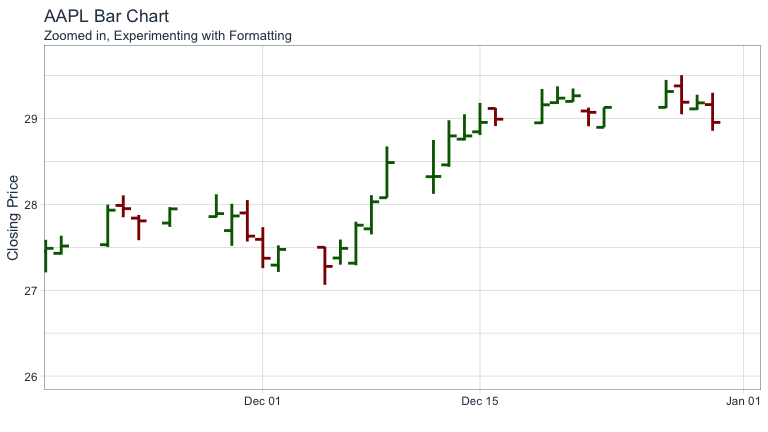## Candlestick Chart

Creating a candlestick chart is very similar to the process with the bar chart. Using `geom_candlestick`, we can insert into the `ggplot` workflow.

``````AAPL %>%
ggplot(aes(x = date, y = close)) +
geom_candlestick(aes(open = open, high = high, low = low, close = close)) +
labs(title = "AAPL Candlestick Chart", y = "Closing Price", x = "") +
theme_tq()``````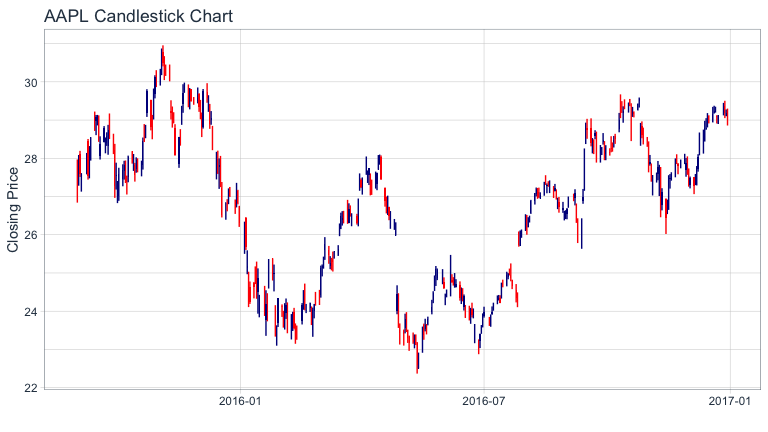We zoom into specific sections using `coord_x_date`.

``````AAPL %>%
ggplot(aes(x = date, y = close)) +
geom_candlestick(aes(open = open, high = high, low = low, close = close)) +
labs(title = "AAPL Candlestick Chart",
subtitle = "Zoomed in using coord_x_date",
y = "Closing Price", x = "") +
coord_x_date(xlim = c(end - weeks(6), end),
c(aapl_range_60_tbl\$min_low, aapl_range_60_tbl\$max_high)) +
theme_tq()``````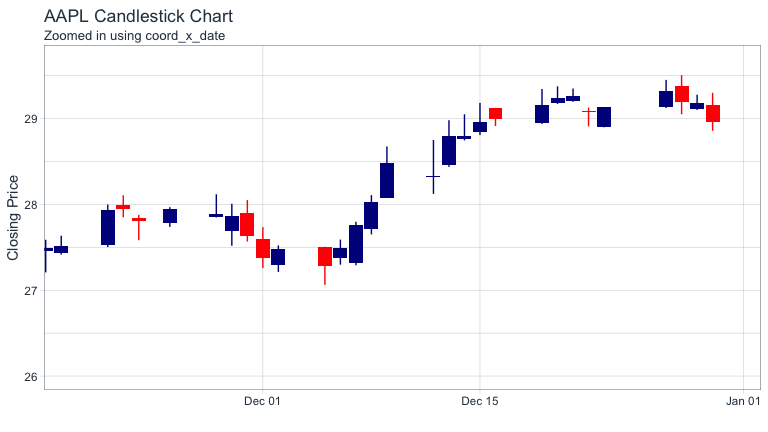The colors can be modified using `colour_up` and `colour_down`, which control the line color, and `fill_up` and `fill_down`, which control the rectangle fills.

``````AAPL %>%
ggplot(aes(x = date, y = close)) +
geom_candlestick(aes(open = open, high = high, low = low, close = close),
colour_up = "darkgreen", colour_down = "darkred",
fill_up  = "darkgreen", fill_down  = "darkred") +
labs(title = "AAPL Candlestick Chart",
subtitle = "Zoomed in, Experimenting with Formatting",
y = "Closing Price", x = "") +
coord_x_date(xlim = c(end - weeks(6), end),
c(aapl_range_60_tbl\$min_low, aapl_range_60_tbl\$max_high)) +
theme_tq()``````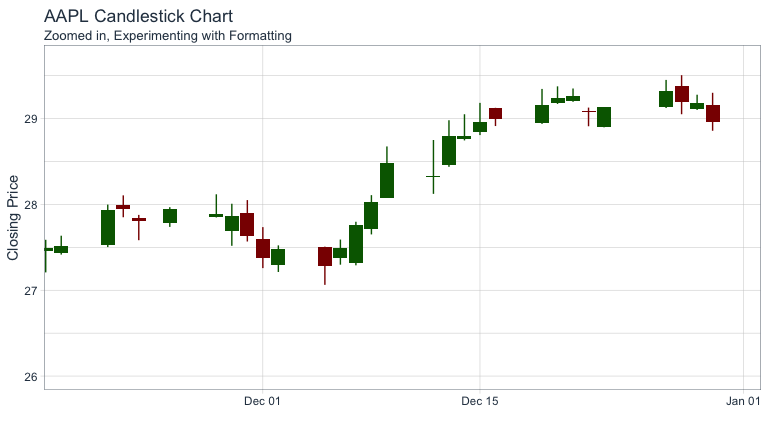## Charting Multiple Securities

We can use `facet_wrap` to visualize multiple stocks at the same time. By adding a `group` aesthetic in the main `ggplot()` function and combining with a `facet_wrap()` function at the end of the `ggplot` workflow, all four “FANG” stocks can be viewed simultaneously. You may notice an odd `filter()` call before the call to `ggplot()`. I’ll discuss this next.

``````start <- end - weeks(6)
FANG %>%
filter(date >= start - days(2 * 15)) %>%
ggplot(aes(x = date, y = close, group = symbol)) +
geom_candlestick(aes(open = open, high = high, low = low, close = close)) +
labs(title = "FANG Candlestick Chart",
subtitle = "Experimenting with Mulitple Stocks",
y = "Closing Price", x = "") +
coord_x_date(xlim = c(start, end)) +
facet_wrap(~ symbol, ncol = 2, scale = "free_y") +
theme_tq()``````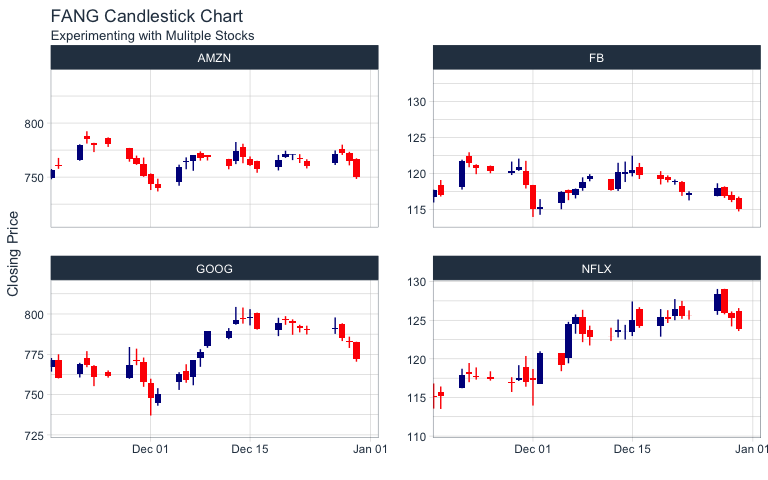A note about out-of-bounds data (or “clipping”), which is particularly important with faceting and charting moving averages:

The `coord_x_date` coordinate function is designed to zoom into specific sections of a chart without “clipping” data that is outside of the view. This is in contrast to `scale_x_date`, which removes out-of-bounds data from the charting. Under normal circumstances clipping is not a big deal (and is actually helpful for scaling the y-axis), but with financial applications users want to chart rolling/moving averages, lags, etc that depend on data outside of the view port. Because of this need for out-of-bounds data, there is a trade-off when charting: Too much out-of-bounds data distorts the scale of the y-axis, and too little and we cannot get a moving average. The optimal method is to include “just enough” out-of-bounds data to get the chart we want. This is why below the FANG data is filtered by date from double the number of moving-average days (`2 * n`) previous to the start date. This yields a nice y-axis scale and still allows us to create a moving average line using `geom_ma`.

``````start <- end - weeks(6)
FANG %>%
filter(date >= start - days(2 * 15)) %>%
ggplot(aes(x = date, y = close, group = symbol)) +
geom_candlestick(aes(open = open, high = high, low = low, close = close)) +
geom_ma(ma_fun = SMA, n = 15, color = "darkblue", size = 1) +
labs(title = "FANG Candlestick Chart",
subtitle = "Experimenting with Mulitple Stocks",
y = "Closing Price", x = "") +
coord_x_date(xlim = c(start, end)) +
facet_wrap(~ symbol, ncol = 2, scale = "free_y") +
theme_tq()``````